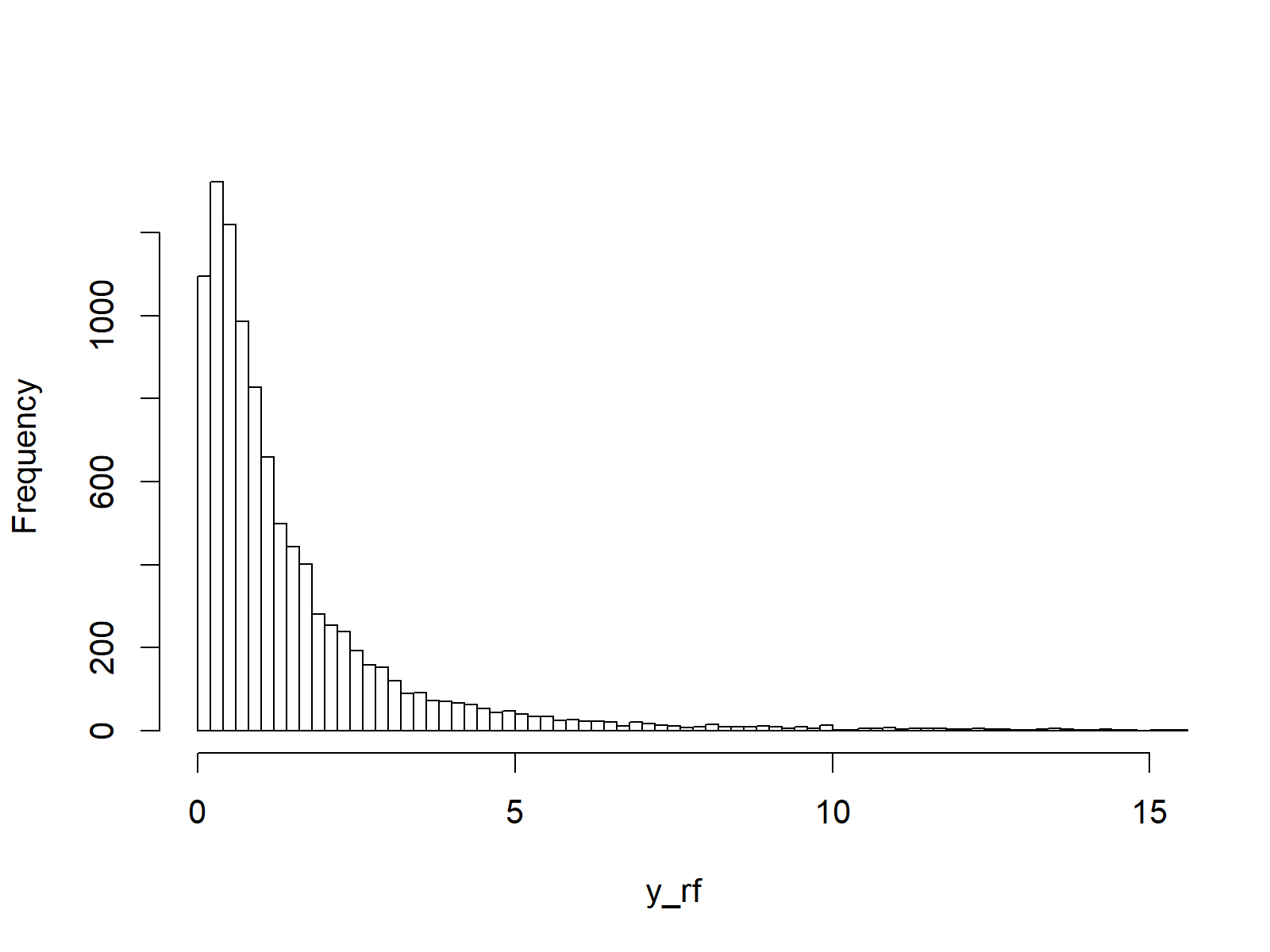# F Distribution in R (4 Examples) | df, pf, qf & rf Functions

In this article, I’ll illustrate how to apply the F distribution functions in the R programming language.

The tutorial will contain this:

Let’s take a look at some R codes in action…

## Example 1: F Density in R (df Function)

In the first example of this tutorial, I’ll explain how to draw a density plot of the F distribution. As a first step, we need to create some input data for the df R function:

`x_df <- seq(0, 20, by = 0.1)                         # Specify x-values for df function`

Now, we can apply the df command to this data…

`y_df <- df(x_df, df1 = 3, df2 = 5)  # Apply df function`

…and use the R plot function to create a graph representing the F density:

`plot(y_df)                                          # Plot df values`Figure 1: Density of F Distribution.

## Example 2: F Cumulative Distribution Function (pf Function)

In the second example, you’ll learn how to draw a cumulative distribution function (CDF) of the F distribution. First, we have to create a vector of quantiles as input:

`x_pf <- seq(0, 20, by = 0.1)                         # Specify x-values for pf function`

Now, we can apply the pf function to this input vector:

`y_pf <- pf(x_pf, df1 = 3, df2 = 5)  # Apply pf function`

We can draw a plot of the output of the pf function as shown below:

`plot(y_pf)                                          # Plot pf values`Figure 2: Cumulative Distribution Function of F Distribution.

## Example 3: F Quantile Function (qf Function)

In the next example, we’ll draw a quantile function plot of the F distribution. First, we need to create a sequence of probabilities:

`x_qf <- seq(0, 1, by = 0.01)                        # Specify x-values for qf function`

Then, we can apply the qf function in order to get the corresponding quantile function values for our input sequence:

`y_qf <- qf(x_qf, df1 = 3, df2 = 5)  # Apply qf function`

And finally, we can draw a plot:

`plot(y_qf)                                          # Plot qf values`Figure 3: Quantile Function of F Distribution.

## Example 4: Simulation of Random Numbers (rf Function)

To generate a set of random numbers, we need to specify a seed and a sample size:

```set.seed(53535)                                          # Set seed for reproducibility
N <- 10000                                               # Specify sample size```

Then, we can use the rf function to draw random numbers according to the F distribution:

```y_rf <- rf(N, df1 = 3, df2 = 5)          # Draw N F-distributed values
y_rf                                                # Print values to RStudio console
# 0.419950200  1.089996596  0.334007814  0.069551464  0.367587034  1.386032672  0.531977959  0.772345591  1.587337077```

The output of the RStudio console illustrates the values created by the rf command. We can now plot these random numbers in a histogram as follows:

```hist(y_rf,                                          # Plot of randomly drawn f density
breaks = 500,
main = "",
xlim = c(0, 15))```Figure 4: Random Numbers Generated According to F Distribution.

## Video, Further Resources & Summary

I have recently published a video on the Statistics Globe YouTube channel, which shows the R programming code of this article. You can find the video below:

You could also have a look at the other articles on probability distributions and the simulation of random numbers in R:

In addition, you may read some of the other articles on my homepage. A selection of tutorials can be found here.

Summary: At this point you should have learned how to use the F functions in the R programming language. Let me know in the comments, if you have any additional questions. Furthermore, don’t forget to subscribe to my email newsletter in order to receive regular updates on new tutorials.

Subscribe to the Statistics Globe Newsletter